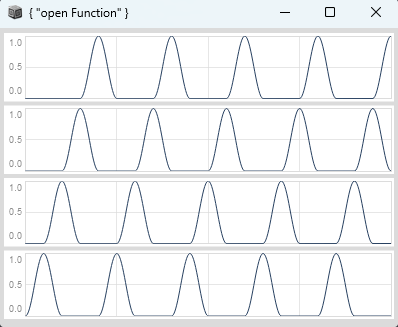# Multichannel phase with LFSaw / Phasor

hey, im creating this multichannel phase by either using LFSaw or Phasor and was wondering why i have to add a little increment to the upper bound of .wrap (here SampleDur.ir) for the Phasor, otherwise the first phase is excluded. I thought wrapping between 0 and 1 would be correct. Are there in general any advantages of using LFSaw vs Phasor or vice versa for this purpose?

(
var multiChannelPhase = { |numChannels, rate|
numChannels.collect{ |i|
var localRate, localPhase, localTrig, hasTriggered;
localRate = rate / numChannels;
// compare with LFSaw
//localPhase = LFSaw.ar(localRate, ((1 - (i / numChannels + 0.5)) * 2)).linlin(-1, 1, 0, 1);
localPhase = (Phasor.ar(DC.ar(0), localRate * SampleDur.ir) + (1 - (i / numChannels))).wrap(0, 1 + SampleDur.ir);
localTrig = HPZ1.ar(localPhase) < 0;
hasTriggered = PulseCount.ar(localTrig) >= 1;
localPhase * hasTriggered;
}.reverse;
};

{
var numChannels = 4;
var overlap = \overlap.kr(2);
var rate = \rate.kr(100);
var phases = multiChannelPhase.(numChannels, rate) * (numChannels / overlap);
IEnvGen.ar(Env([0, 1, 0], [0.5, 0.5], \sin), phases);
}.plot(0.2);
)1 Like

Phasor’s initial sample is 0.

For channel i = 0, you’re adding 1 – + output value is 1.

.wrap(0, 1) wraps this back around to 0.

So HPZ1 begins with a rising signal. But it detects a trigger when the signal drops. This does not happen at the beginning of the synth.

The .wrap(0, 1 + SampleDur.ir) approach hacks the math so that this initial 1 stays 1 – then the second sample is lower, causing a trigger. But the wrap cycle now disagrees with the Phasor cycle – in the other channels, (Phasor + fractional_offset).wrap(0, 1 + SampleDur.ir) might be slightly different from expected.

Perhaps a better solution would be to subtract SampleDur from the Phasor before wrapping, so that the first channel begins at the end of its cycle, and then you get the early trigger, and the phase relationships between the channels will all be intact.

localPhase = (Phasor.ar(DC.ar(0), localRate * SampleDur.ir) + (1 - (i / numChannels)) - SampleDur.ir).wrap(0, 1);


hjh

thanks for the explanation, thats it!